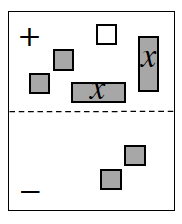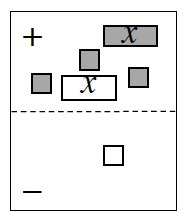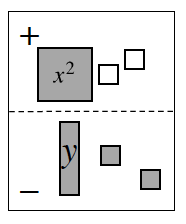### Home > CCA > Chapter A > Lesson A.1.6 > ProblemA-61

A-61.

Find a simplified algebraic expression for each expression mat below.1.The circled tiles can be removed, since they make zero pairs. What is an expression for the remaining tiles?

$2x − 1$

Use the eTool below to find the simplified algebraic expression.
Click on the link at right for the full eTool version: CCA A-61a HW eTool

1.On this mat, both $x$-tiles in the ($+$) region can be removed, since they make a zero pair. Next, flip the $−1$ unit tile in the ($−$) region to the ($+$) region, and then simplify.

Use the eTool below to find the simplified algebraic expression.
Click on the link at right for the full eTool version: CCA A-61b HW eTool

1.Unlike in parts (a) and (b), this problem has no zero pairs. However, here is an un-simplified expression to get you started.
$x^{2} + \left(−1\right) + \left(−1\right) − \left(y + 1 + 1\right)$
Can you simplify that expression?

Use the eTool below to find the simplified algebraic expression.
Click on the link at right for the full eTool version: CCA A-61c HW eTool